Question

# calculating the ph of a weak acid titrated with a strong base An analytical chemist is...

calculating the ph of a weak acid titrated with a strong base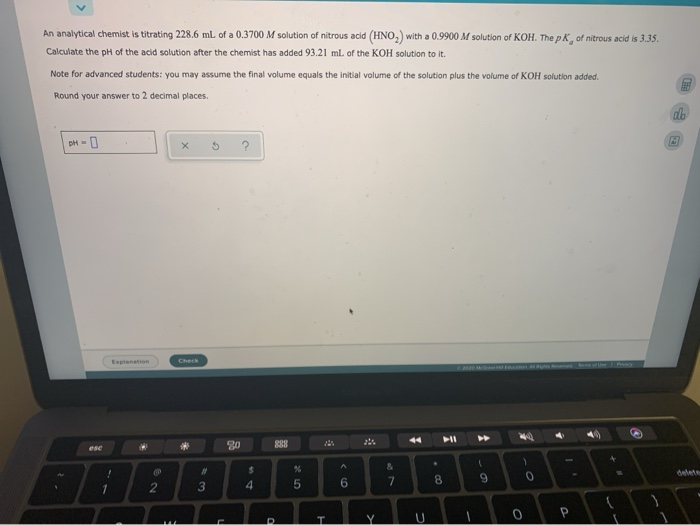An analytical chemist is titrating 228.6 ml. of a 0.3700 M solution of nitrous acid (HNO,) with a 0.9900 M solution of KOH. The pk of nitrous acid is 3.35. Calculate the pH of the acid solution after the chemist has added 93.21 ml of the KOH solution to it. Note for advanced students: you may assume the final volume equals the initial volume of the solution plus the volume of KOH solution added. Round your answer to 2 decimal places. | oh 1 x S ?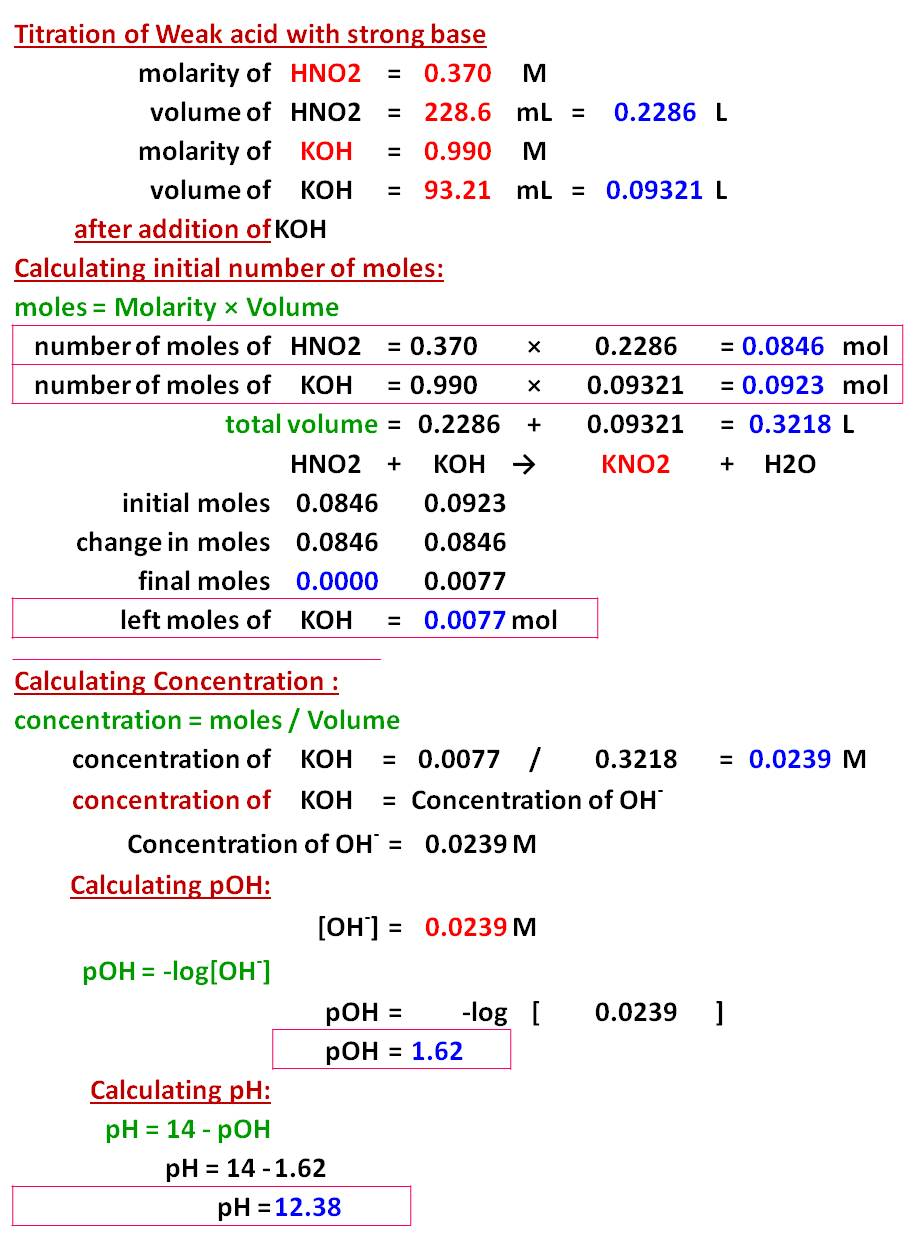#### Earn Coins

Coins can be redeemed for fabulous gifts.

Similar Homework Help Questions
• ### Calculating the ph of a weak acid bitrated with a strong base An analytical Chemist is...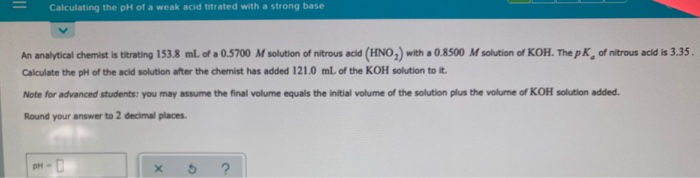Calculating the ph of a weak acid bitrated with a strong base An analytical Chemist is titrating 153.8 ml. of a 0.5700 M solution of nitrous acid (HNO,) with a 0.8500 M solution of KOH. The pk of nitrous acid is 3.35. Calculate the pH of the acid solution after the chemist has added 121.0 ml. of the KOH solution to it. Note for advanced students: you may assume the final volume equals the initial volume of the solution plus...

• ### - Calculating the pH of a weak acid titrated with a strong base An analytical chemist is titrating 123.0 ml. of a 0...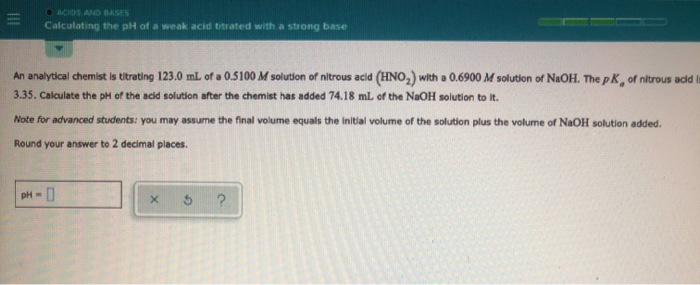- Calculating the pH of a weak acid titrated with a strong base An analytical chemist is titrating 123.0 ml. of a 0.5100 M solution of nitrous acid (HNO,) with a 0.6900 M solution of NaOH. The pk of nitrous add 3.35. Calculate the pH of the acid solution after the chemist has added 74.18 ml of the NaOH solution to it. Note for advanced students you may assume the final volume equals the initial volume of the solution plus...

• ### calculating the ph of a weak base titrated with a strong acid An analytical chemist is...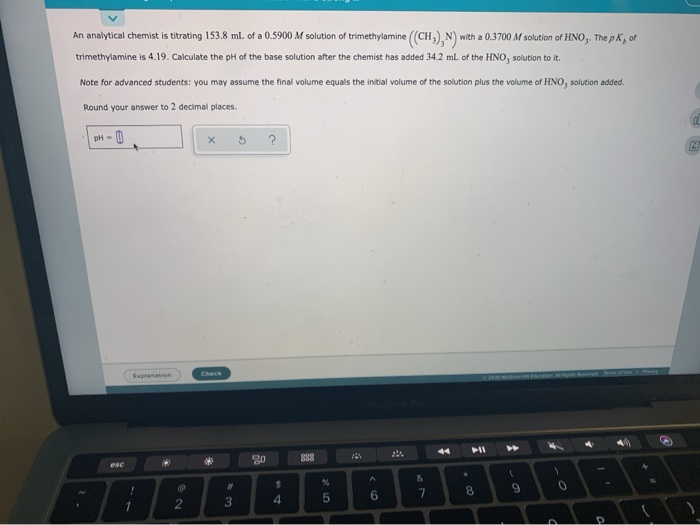calculating the ph of a weak base titrated with a strong acid An analytical chemist is titrating 153.8 ml of a 0.5900 M solution of trimethylamine (CH), N) with a 0.3700 M solution of HNOJ. The pl, of trimethylamine is 4.19. Calculate the pH of the base solution after the chemist has added 34.2 mL of the HNO, solution to it Note for advanced students: you may assume the final volume equals the initial volume of the solution plus the...

• ### Calculating the ph of a weak acid bitrated with a strong base An analytical Chemist is...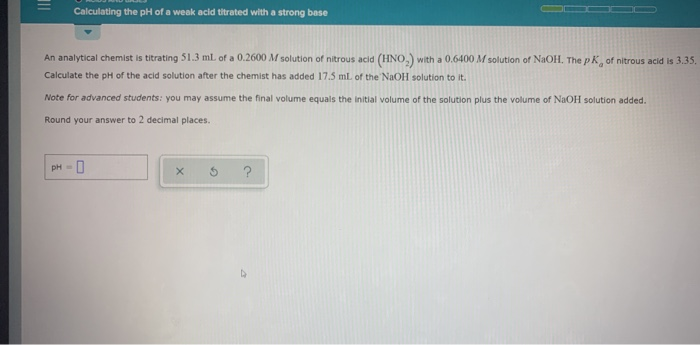Calculating the ph of a weak acid bitrated with a strong base An analytical Chemist is titrating 51.3 ml of a 0.2600 M solution of nitrous acid (HNO.) with a 0.6400 M solution of NaOH. The pk of nitrous acid is 3.35 Calculate the pH of the acid solution after the chemist has added 17.5 mL of the NaOH solution to it. Note for advanced students: you may assume the final volume equals the initial volume of the solution plus...

• ### calculating the ph of a weak acid titrated with a strong base An analytical chemist is...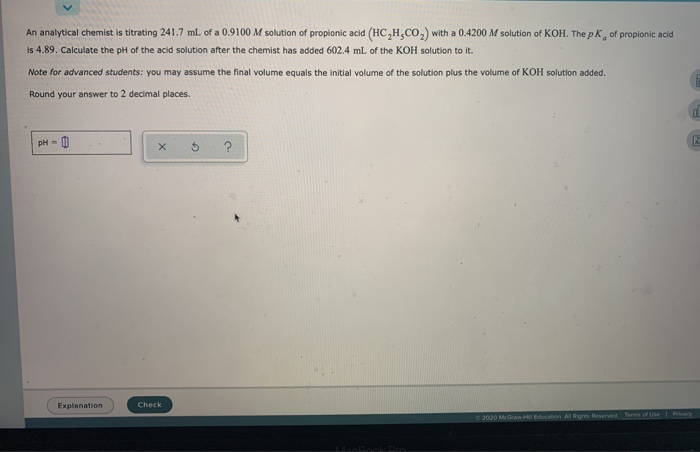calculating the ph of a weak acid titrated with a strong base An analytical chemist is titrating 241.7 ml of a 0.9100 M solution of propionic acid (HCH.CO) with a 0.4200 M solution of KOH. The pk of propionic acid is 4.89. Calculate the pH of the acid solution after the chemist has added 602.4 ml of the KOH solution to it. Note for advanced students: you may assume the final volume equals the initial volume of the solution plus...

• ### calculating the ph of a weak acid titrated with a strong base An analytical chemist is...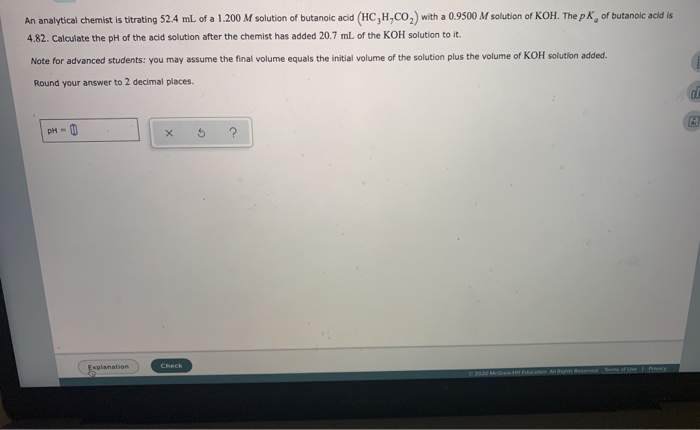calculating the ph of a weak acid titrated with a strong base An analytical chemist is titrating 52.4 ml of a 1.200 M solution of butanoic acid (HC,H,CO) with a 0.9500 M solution of KOH. The pK, of butanoic acid is 4.82. Calculate the pH of the acid solution after the chemist has added 20.7 mL of the KOH solution to it. Note for advanced students: you may assume the final volume equals the initial volume of the solution plus...

• ### calculating the ph of a weak base titrated with a strong acid An analytical Chemist is...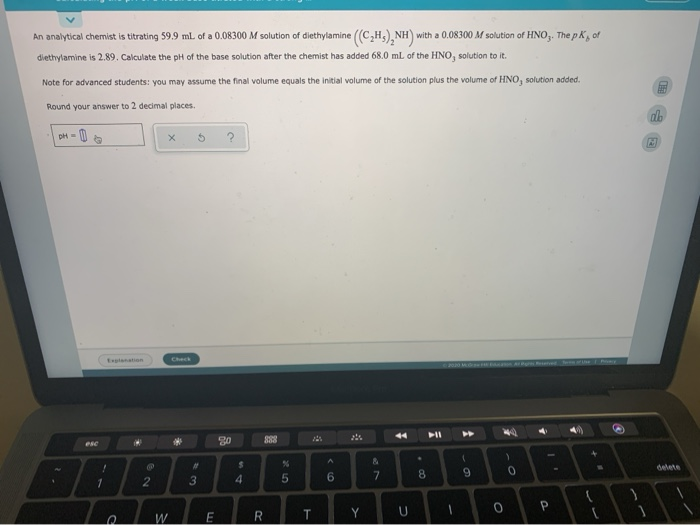calculating the ph of a weak base titrated with a strong acid An analytical Chemist is titrating 59.9 ml. of a 0.08300 M solution of diethylamine (CH), NH with a 0.08300 M solution of HNO,. The pl, of diethylamine is 2.89. Calculate the pH of the base solution after the chemist has added 68.0 mL of the HNO, solution to it. Note for advanced students: you may assume the final volume equals the initial volume of the solution plus the...

• ### Calculating the pit of a weak base titrated with a strong acid An analytical chemist is...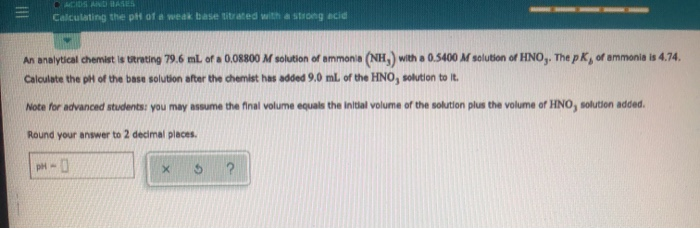Calculating the pit of a weak base titrated with a strong acid An analytical chemist is troting 79.6 ml. of a 0.08800 M solution of ammonia (NH,) with a 0.5400 M solution of HNO,. The pK, of ammonia is 4.74. Calculate the pH of the base solution after the chemist has added 9.0 mL of the HNO, solution to it. Note for advanced students: you may assume the final volume equals the initial volume of the solution plus the volume...

• ### Calculating the pH of a weak acid titrated with a strong base An analytical chemist is...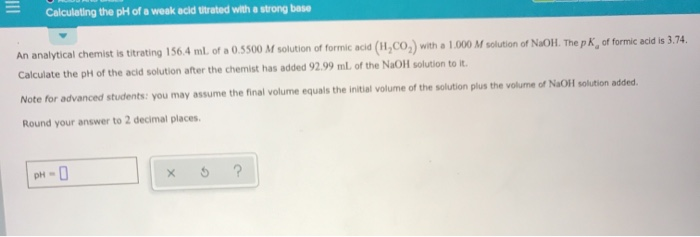Calculating the pH of a weak acid titrated with a strong base An analytical chemist is titrating 156.4 ml. of a 0.5500 M solution of formic acid (H,CO,) with a 1.000 M solution of NaOH. The p K, of formic acid is 3.74. Calculate the pH of the acid solution after the chemist has added 92.99 mL of the NaOH solution to it. Note for advanced students: you may assume the final volume equals the initial volume of the solution...

• ### ACIDS AND BASES Calculating the pH of a weak base titrated with a strong An analytical...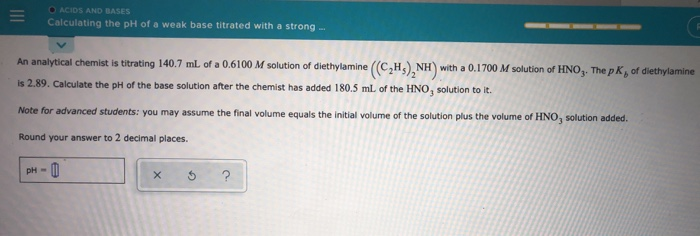ACIDS AND BASES Calculating the pH of a weak base titrated with a strong An analytical Chemist is titrating 140.7 mL of a 0.6100 M solution of diethylamine (CH), NH with a 0.1700 M solution of HNO. The pk, of diethylamine is 2.89. Calculate the pH of the base solution after the chemist has added 180.5 ml of the HNO, solution to it. Note for advanced students: you may assume the final volume equals the initial volume of the solution...